# SV Get Data Subset VI

## Sound and Vibration Measurement Help

Edition Date: March 2018
Part Number: 372416L-01
View Product InfoLabVIEW 2018 Sound and Vibration Toolkit HelpLabVIEW 2019 Sound and Vibration Toolkit Help

Owning Palette: Common Utilities VIs

Requires: Sound and Vibration Toolkit

Returns a portion of the waveform of the start index and length values. When you wire a waveform to this VI, the waveform automatically resizes to display start index inputs for the waveform.

Use the pull-down menu to select an instance of this VI.

 Select an instance svc_Get Array Subset (Index)svc_Get Waveform Subset (Index)

## svc_Get Array Subset (Index)Y specifies the array for which you want to retrieve a subset.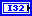start index (0) specifies the data element where you want to start acquiring a subset. start index must be less than or equal to the length–1. start index can be positive or negative. Positive values of start index move the subset index up from zero, while negative values move the subset back from the end of the array. If start index is negative, the actual start index equals length + start index.length (-1 = all) specifies the number of samples to retrieve. If length is negative, the function returns the array from the actual start index to the end of the array.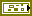error in describes error conditions that occur before this node runs. This input provides standard error in functionality.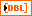Y subset returns the array subset.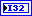actual length returns the actual number of elements retrieved from the input array.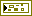error out contains error information. This output provides standard error out functionality.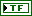end reached indicates whether the specified subset has reached the end of the input array.

## svc_Get Waveform Subset (Index)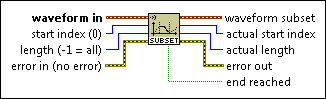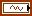waveform in specifies the waveform for which you want to retrieve a subset.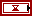t0 specifies the start time of the waveform.dt specifies the time interval in seconds between data points in the waveform.Y specifies the data values of the waveform.start index (0) specifies the data element where you want to start acquiring a subset. start index must be less than or equal to the length–1. start index can be positive or negative. Positive values of start index move the subset index up from zero, while negative values move the subset back from the end of the array. If start index is negative, the actual start index equals length + start index.length (-1 = all) specifies the number of samples to retrieve. If length is negative, the function returns the array from the actual start index to the end of the array.error in describes error conditions that occur before this node runs. This input provides standard error in functionality.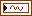waveform subset returns the waveform subset.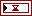t0 returns the start time of the waveform.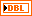dt returns the time interval in seconds between data points in the waveform.Y returns the data values of the waveform.actual length returns the actual number of elements retrieved from the input array.error out contains error information. This output provides standard error out functionality.end reached indicates whether the specified subset has reached the end of the input array.